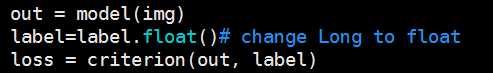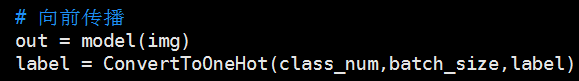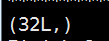# How to get the partial derivative of the output data with the middle layer data

In a MLP neural network, I want to get partial derivative of the output data with the middle layer data. such as, as shown below:,i need the partial derivative of Etotal with neto1. i find register_backward_hook can get this value, but i fail to use. below is my code. i do not know the error

Does that not work for you? What happens?

first, thanks for reply. This code is work, but i don’t know whether the obtained values of model.layer3.register_backward_hook(hook) are partial derivative of Etotal and neto. Second, I want to use nn.MSELoss() replace nn.CrossEntropyLoss(), so, the criterion= nn.CrossEntropyLoss() change to criterion= nn.MSELoss(size_average = False).this code proceed with error below:

then, i add line code:but, there also has error:

for this error, i really don’t know how to solve this problem or how to use MSELoss in my program
I know the ‘’target‘’ of MSELoss is ：Presumably `label` contains the labels as class identifier numbers, not as a 1-hot encoding.

1 Like

thanks for replay. according above contents, I change my code ,as below:but there is a error, do you know how to solve?

It looks like `label` is a Variable, not a Tensor. Try this…

``````label_onehot.scatter_(1, label.data, 1.0)
``````

We need to check the size of `label`. What does `print(label.size())` show?this is the result. I really appreciate your help.

I assume 32 is your batch size.

Try this…

``label_onehot.scatter_(1, label.data.unsqueeze(dim=1), 1.0)``

there is another problem,sorry.

As you say, batch_size=32

The one_hot labels are Tensors not Variables, you need to wrap them in a Variable.
e.g. just after the line we worked on previously.

``return Variable(label_onehot)``

thanks , this is work ,but my code have another error. i think i need myself to do first, if i can’t solve, i will ask you, thanks, thanks,thanks

my program is work, thank you very much

1 Like